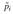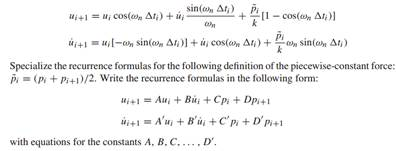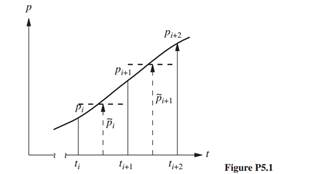### Create an Account

Already have account?

### Forgot Your Password ?

Home / Questions / In Section 52 we developed recurrence formulas for numerical solution of the equation of m...

# In Section 52 we developed recurrence formulas for numerical solution of the equation of motion of a linear SDF system based on linear interpolation of the forcing function pt over each time step

In Section 5.2 we developed recurrence formulas for numerical solution of the equation of motion of a linear SDF system based on linear interpolation of the forcing function p(t) over each time step. Develop a similar procedure using a piecewise-constant representation of the forcing function wherein the value of the force in the interval ti to ti+1 is a constant equal to(Fig. P5.1). Show that the recurrence formulas for the response of an undamped system areJun 11 2020 View more View Less

#### Answer (Solved)Subscribe To Get Solution